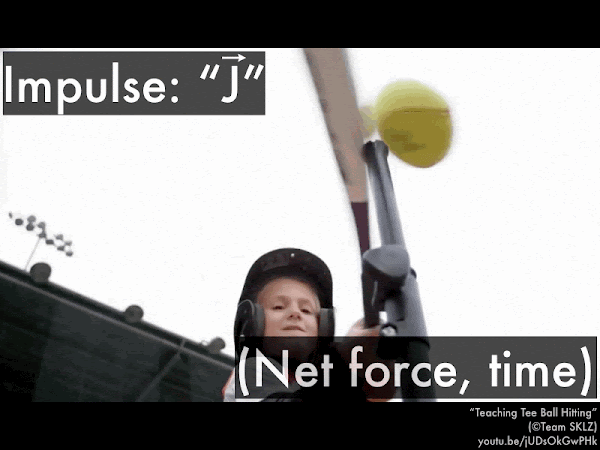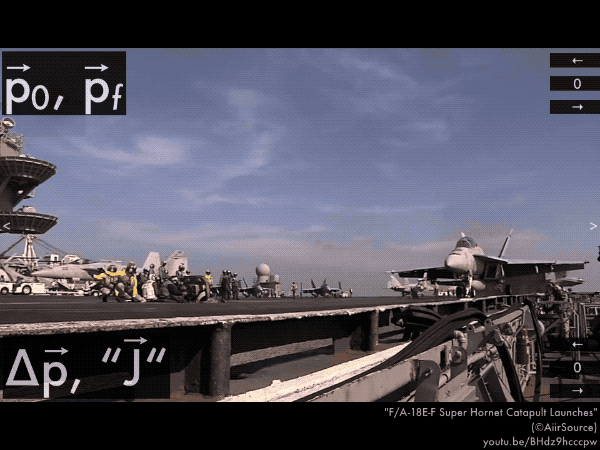## 20171011

### Online reading assignment: impulse and momentum

Physics 205A, fall semester 2017
Cuesta College, San Luis Obispo, CA

Students have a bi-weekly online reading assignment (hosted by SurveyMonkey.com), where they answer questions based on reading their textbook, material covered in previous lectures, opinion questions, and/or asking (anonymous) questions or making (anonymous) comments. Full credit is given for completing the online reading assignment before next week's lecture, regardless if whether their answers are correct/incorrect. Selected results/questions/comments are addressed by the instructor at the start of the following lecture.

The following questions were asked on reading textbook chapters and previewing a presentation on impulse and momentum.Selected/edited responses are given below.

Describe what you understand from the assigned textbook reading or presentation preview. Your description (2-3 sentences) should specifically demonstrate your level of understanding.
"This is another new approach to connect forces with changes in motion. This new approach does not involve Newton's laws but instead uses impulse and momentum (both are vectors)."

"Momentum is clicking very well for me. I understand that momentum is mass times velocity. It is also a vector quantity."

"An object's momentum is its mass and velocity combined. Impulse is the change in momentum, which is the average net force over an elapsed time."

"Impulse causes a change in momentum, and impulse is defined by the average net force exerted on an object multiplied by the contact time, so how long that force was exerted on the object. Momentum is mass times velocity."

"Impulse is equal to the change in momentum. Impulse is also a vector quantity and has the same direction as the average force, and is force times ∆t."

"Impulse is related to momentum because impulse is equal to the change of momentum."

"The impulse must have the same direction as the change in momentum, and so it must also point to the right if ∆p is pointing or going to the right."

"If a force is applied on an object for a given amount of time, that object feels an impulse."

Describe what you found confusing from the assigned textbook reading or presentation preview. Your description (2-3 sentences) should specifically identify the concept(s) that you do not understand.
"After reading this section, I think I still need additional help understanding the whole impulse concept."

"Most of this is confusing. Mainly how to calculate impulse of an object. I'm not really sure still what impulse is or when to use it."

"I have a hard time visualizing how impulse works. I guess until now I was just thinking about impulse and momentum as the same thing?"

"Wrapping my head around the impulse-momentum theorem."

"I am confused by impulse but I am okay with momentum."

"The units of impulse because I find them nonintuitive. I think it's more of a personal conceptual issue but sometimes units are nonintuitive."

"Nothing was necessarily confusing, I just need practice with the new round of formulas."

"I found this pretty simple."For the child hitting the tee ball with a bat, if the bat is swung such that it exerts the same net force on the tee ball for a longer time (by giving the bat more "follow-through"), the impulse on the tee ball will be __________, and the change in momentum of the tee ball will be:
 less; less.  less; greater. ***  greater; less. *******  greater; greater. **************************  (Unsure/lost/guessing/help!) *For a golf ball initially at rest, and then has a speed of 97 m/s after being hit by a golf club, indicate the horizontal directions (+/– signs) for the various impulse-momentum theorem quantities. (Only correct responses shown.)
Golf ball's initial momentum p0: no direction (0). [89%]
Golf ball's final momentum pf: to the right (+). [95%]
Golf ball's initial-to-final change in momentum ∆p: to the right (+). [86%]
Golf club's impulse "J" on the golf ball: to the right (+). [76%]For this catapult-launched F/A-18E-F Super Hornet taken from rest to a final speed of 74 m/s, indicate the directions (+/– signs) for the various impulse-momentum theorem quantities. (Only correct responses shown.)
Super Hornet's initial momentum p0: no direction (0). [%]
Super Hornet's final momentum pf: to the left (–). [32%]
Super Hornet's initial-to-final change in momentum ∆p: to the left (–). [19%]
Catapult's impulse "J" on the Super Hornet: to the left (–). [22%]For this Ford Ranger, hitting a crash barrier with a speed of 11.0 m/s, and then rebounding off the crash barrier with a speed of 2.2 m/s, indicate the directions (+/– signs) for the various impulse-momentum theorem quantities. (Only correct responses shown.)
Ford Ranger's initial momentum p0: to the right (+). [73%]
Ford Ranger's final momentum pf: to the left (–). [78%]
Ford Ranger's initial-to-final change in momentum ∆p: to the left (–). [49%]
Crash barrier's impulse "J" on the Ford Ranger: to the left (–). [51%]
Ask the instructor an anonymous question, or make a comment. Selected questions/comments may be discussed in class.
"Could you please go over the above examples in class?"

"Please review the Ford Ranger problem!"

"Going over the above examples would be extremely helpful. Thank you for the extra examples and explanations in class lately!"

"If two objects moving in opposite directions hit each other, how do we measure the impulse?" (You just need to know the mass of each car, and each car's initial and final velocities. Accident reconstruction experts can look up the mass of a vehicle, and then deduce the initial and final velocities of each car by looking at various clues such as length of tire skid marks, vehicle sensor recordings, etc.)

"Great job so far! I'm really enjoying your class."

"Sorry, I have been loaded with research and studying lately. I don't mean to skip your assignments, but I appreciate that you take into account busy schedules and let that slide. Thank you." (You still get credit for completing as much as you can on these reading assignments, even if it's just touching bases with me.)

"Will there be questions on the midterm with similar format as the homework?" (Yes, since many of these questions are taken from previous semesters' midterms. We'll start reviewing for the midterm today.)